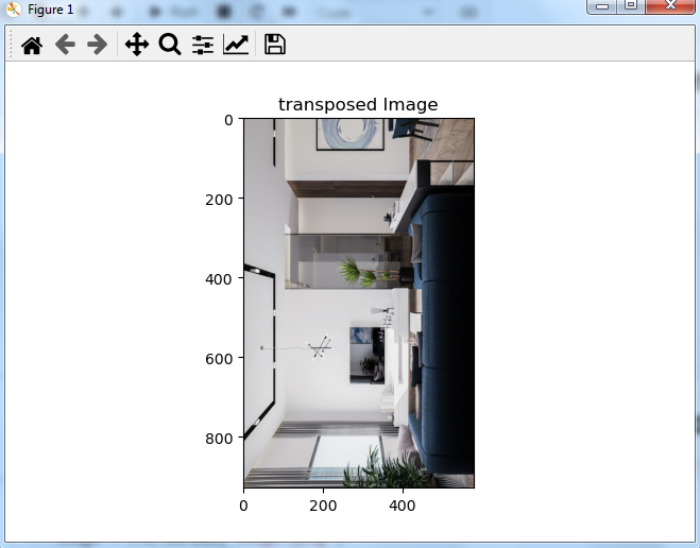# How to perform image transpose using OpenCV Python?

In OpenCV, the image is NumPy ndarray. The image transpose operation in OpenCV is performed as the transpose of a NumPy 2D array (matrix). A matrix is transposed along its major diagonal. A transposed image is a flipped image over its diagonal. We use cv2.transpose() to transpose an image.

## Steps

We could use the following steps to transpose an input image −

• Import required libraries OpenCV and Matplotlib. Make sure you have already installed them.

• Read the input image using cv2.imread(). Specify the full path of the image. Assign the image to a variable img.

• Transpose the input image using cv2.transpose(img). It transposes the pixel values.

• Display the transposed image.

Let's look at the example below for a clear understanding.

## Example

In this Python code, we transpose the input image −

# import required libraries
import cv2
import matplotlib.pyplot as plt

# transpose the input image
image = cv2.transpose(img)

# Displaying the image
plt.imshow(cv2.cvtColor(image, cv2.COLOR_BGR2RGB))
plt.title("transposed Image")
plt.show()


We will use the following image as the input file for this program −When you execute the above code, it will produce the following outputNotice that the transposed image is flipped over its major diagonal.

Updated on: 05-Dec-2022

1K+ Views